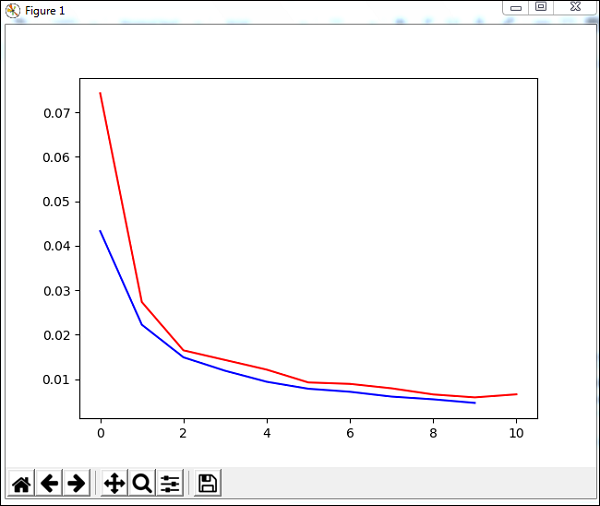# PyBrain - Datasets Types

Datasets are data to be given to test, validate and train on networks. The type of dataset to be used depends on the tasks that we are going to do with machine learning. We are going to discuss the various dataset types in this chapter.

We can work with the dataset by adding the following package −

pybrain.dataset


## SupervisedDataSet

SupervisedDataSet consists of fields of input and target. It is the simplest form of a dataset and mainly used for supervised learning tasks.

Below is how you can use it in the code −

from pybrain.datasets import SupervisedDataSet


The methods available on SupervisedDataSet are as follows −

This method will add a new sample of input and target.

### splitWithProportion(proportion=0.10)

This will divide the datasets into two parts. The first part will have the % of the dataset given as input, i.e., if the input is .10, then it is 10% of the dataset and 90% of data. You can decide the proportion as per your choice. The divided datasets can be used for testing and training your network.

copy() − Returns a deep copy of the dataset.

clear() − Clear the dataset.

### saveToFile(filename, format=None, **kwargs)

Save the object to file given by filename.

### Example

Here is a working example using a SupervisedDataset −

testnetwork.py

from pybrain.tools.shortcuts import buildNetwork
from pybrain.structure import TanhLayer
from pybrain.datasets import SupervisedDataSet
from pybrain.supervised.trainers import BackpropTrainer

# Create a network with two inputs, three hidden, and one output
nn = buildNetwork(2, 3, 1, bias=True, hiddenclass=TanhLayer)

# Create a dataset that matches network input and output sizes:
norgate = SupervisedDataSet(2, 1)

# Create a dataset to be used for testing.
nortrain = SupervisedDataSet(2, 1)

# Add input and target values to dataset
# Values for NOR truth table

# Add input and target values to dataset
# Values for NOR truth table

#Training the network with dataset norgate.
trainer = BackpropTrainer(nn, norgate)

# will run the loop 1000 times to train it.
for epoch in range(1000):
trainer.train()
trainer.testOnData(dataset=nortrain, verbose = True)


### Output

The output for the above program is as follows −

python testnetwork.py

C:\pybrain\pybrain\src>python testnetwork.py
Testing on data:
('out: ', '[0.887 ]')
('correct:', '[1 ]')
error: 0.00637334
('out: ', '[0.149 ]')
('correct:', '[0 ]')
error: 0.01110338
('out: ', '[0.102 ]')
('correct:', '[0 ]')
error: 0.00522736
('out: ', '[-0.163]')
('correct:', '[0 ]')
error: 0.01328650
('All errors:', [0.006373344564625953, 0.01110338071737218, 0.005227359234093431
, 0.01328649974219942])
('Average error:', 0.008997646064572746)
('Max error:', 0.01328649974219942, 'Median error:', 0.01110338071737218)


## ClassificationDataSet

This dataset is mainly used to deal with classification problems. It takes in input, target field and also an extra field called "class" which is an automated backup of the targets given. For example, the output will be either 1 or 0 or the output will be grouped together with values based on input given., i.e., it will fall in one particular class.

Here is how you can use it in the code −

from pybrain.datasets import ClassificationDataSet
Syntax
// ClassificationDataSet(inp, target=1, nb_classes=0, class_labels=None)


The methods available on ClassificationDataSet are as follows −

addSample(inp, target) − This method will add a new sample of input and target.

splitByClass() − This method will give two new datasets, the first dataset will have the class selected (0..nClasses-1), the second one will have remaining samples.

_convertToOneOfMany() − This method will convert the target classes to a 1-of-k representation, retaining the old targets as a field class

Here is a working example of ClassificationDataSet.

### Example

from sklearn import datasets
import matplotlib.pyplot as plt
from pybrain.datasets import ClassificationDataSet
from pybrain.utilities import percentError
from pybrain.tools.shortcuts import buildNetwork
from pybrain.supervised.trainers import BackpropTrainer
from pybrain.structure.modules import SoftmaxLayer
from numpy import ravel
X, y = digits.data, digits.target
ds = ClassificationDataSet(64, 1, nb_classes=10)

for i in range(len(X)):
test_data_temp, training_data_temp = ds.splitWithProportion(0.25)
test_data = ClassificationDataSet(64, 1, nb_classes=10)

for n in range(0, test_data_temp.getLength()):
training_data = ClassificationDataSet(64, 1, nb_classes=10)

for n in range(0, training_data_temp.getLength()):
test_data._convertToOneOfMany()
training_data._convertToOneOfMany()
net = buildNetwork(training_data.indim, 64, training_data.outdim, outclass=SoftmaxLayer)
trainer = BackpropTrainer(
net, dataset=training_data, momentum=0.1,learningrate=0.01,verbose=True,weightdecay=0.01
)
trnerr,valerr = trainer.trainUntilConvergence(dataset=training_data,maxEpochs=10)
plt.plot(trnerr,'b',valerr,'r')
plt.show()
trainer.trainEpochs(10)
print('Percent Error on testData:',percentError(trainer.testOnClassData(dataset=test_data), test_data['class']))


The dataset used in the above example is a digit dataset and the classes are from 0-9, so there are 10 classes. The input is 64, target is 1 and classes, 10.

The code trains the network with the dataset and outputs the graph for training error and validation error. It also gives the percent error on testdata which is as follows −

### OutputTotal error: 0.0432857814358
Total error: 0.0222276374185
Total error: 0.0149012052174
Total error: 0.011876985318
Total error: 0.00939854792853
Total error: 0.00782202445183
Total error: 0.00714707652044
Total error: 0.00606068893793
Total error: 0.00544257958975
Total error: 0.00463929281336
Total error: 0.00441275665294
('train-errors:', '[0.043286 , 0.022228 , 0.014901 , 0.011877 , 0.009399 , 0.007
822 , 0.007147 , 0.006061 , 0.005443 , 0.004639 , 0.004413 ]')
('valid-errors:', '[0.074296 , 0.027332 , 0.016461 , 0.014298 , 0.012129 , 0.009
248 , 0.008922 , 0.007917 , 0.006547 , 0.005883 , 0.006572 , 0.005811 ]')
Percent Error on testData: 3.34075723830735